# Calorimetry

Calorimetry is the measurement of the quantity of heat exchanged. For example, if the energy from an exothermic chemical reaction is absorbed in a container of water, the change in temperature of the water provides a measure of the amount of heat added. Calorimeters are used to determine the energy content of foods by burning the foods in an oxygen atmosphere and measuring the energy yield in terms of the increase in temperature of the calorimeter. Calorimeters can also be used to measure the specific heat of a substance.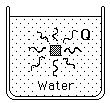The materials involved in the calorimetry are modeled here as a volume of water, a source of heat which is characterized by its equivalent water mass, and the container or calorimeter with its mass and specific heat. Thermal equilibrium is assumed after the experiment so that the temperature change can be used to calculate the energy released. Mass of water = gm Equivalent water mass of heat source = gm. Mass of calorimeter = gm. Specific heat of calorimeter = cal/gm C = joule/gm C.

If the calorimeter increases in temperature by C, then the amount of heat released is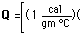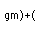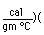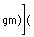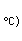Q = calories = joules
Index

Heat transfer concepts

Heat transfer examples

Thermal equilibrium

 HyperPhysics***** Thermodynamics R Nave
Go Back